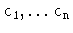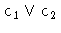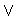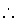Next: Conclusions and Further Reading Up: Pragmatics Previous: Controlling the Control

# Complex Constraints

Some languages include specialized primitives which set up complex systems of equations for solving problems which appear often (for example, scheduling tasks subject to a maximum instantaneous availability of resources). These primitives may also perform enumeration, often taking into account how the constraints have been set up, and trying to work out a solution as efficiently as possible.

Other complex constraints allow expressing simpler constraints as a special case. They allow also setting up in a simple, compact expression, constraints which would otherwise be verbose to construct. For example, the complex constraint #(L, [c <TT>1, ..., c <TT>n], U), called cardinality operator, states that the number of true constraints in c <TT>1, ..., c <TT>n is, at least L, and, a most U. The numbers L and U act as parameters which change the effect of the constraint:

• If L and U are equal to n, then all constraints must be true: this boils down to the conjunction of.
• If L and U are equal to one, then only one of the constraints can (and must) be true.
• if L and U are both zero, then none of the constraints can be true: this is tantamount to requiring the conjunction of the negated constraints to be true.

A specially interesting constraint is the so-called disjunctive constraint:expresses that at least one of these constraints ( c1 or c2) is true; this is a particular case of the cardinality operator, when L is equal to one and U is equal to 2. It can also be written using a predicate with several clauses. However, disjunctive constraints may sometimes have advantages. The following predicate expresses that a number does not belong to the interval [-1, 1]:

n1(X):- X > 1.
n1(X):- X < -1.


If this predicate is called with its argument not definitely inside or outside that interval, and due to the constraint semantics, the execution does not actually select among the different clauses immediately, but a choicepoint is set instead, a constraint added, and the execution is continued. Backtracking may happen later if the alternative chosen was not the right one. A disjunctive constraint such as

n1(X):- X > 1X < -1.

will add the disjunctive constraint, and execution will continue as long as any of the disjuncted constraints hold. In this case, a possibly expensive backtracking would have been avoided.Next: Conclusions and Further Reading Up: Pragmatics Previous: Controlling the Control
MCL
1998-12-03